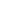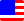Documents  >  Tutorial  >  Priority 2
Priority 2 operators

It describes the following operators with priority 2.

 . x.y Dot operator ++ x++ Postfix ++ operator -- x-- Postfix -- operator

They are unary operators placed after the operand.

Dot operator

Postfix ++ operator

Postfix -- operator

Dot operator

The dot operator is an operation in order to call a member of the operand.

This operator call a member of the instance that is returned by the left expression of the operator. The member is specified by the right expression of the operator.

int i = new int(10); // 10

string str = i.ToString(); // "10"

The variable i of the int class is instructed to call the ToString () method.

Postfix ++ operator

The postfix ++ operator returns a new instance with the same value as the operand and then increments the value of the operand by 1.

The operand must be one of the int, long, or real classes. Using the postfix ++ operator on any other class will throw an exception.

Even if the operand is the maximum value for that class, the postfix ++ operator will not cause an overflow. It will be the minimum value of the class.

int i = 10;

int j = i++; // j is 10. i is 11.

If the operand returns the proxy class, the entity of the proxy is automatically used. The result is the same as calling the Entity getter of the proxy class.

int i = 10;

proxy pro = new proxy(i);

int j = 10 + pro++; // j is 20. i is incremented.

Postfix -- operator

The postfix -- operator returns a new instance with the same value as the operand and then decrements the value of the operand by 1.

The operand must be one of the int, long, or real classes. Using the postfix -- operator on any other class will throw an exception.

Even if the operand is the minimum value for that class, the postfix -- operator will not cause an overflow. It will be the maximum value of the class.

int i = 10;

int j = i--; // j is 10. i is 9.

If the operand returns the proxy class, the entity of the proxy is automatically used. The result is the same as calling the Entity getter of the proxy class.

int i = 10;

proxy pro = new proxy(i);

int j = 10 + pro--; // j is 20. i is decremented.